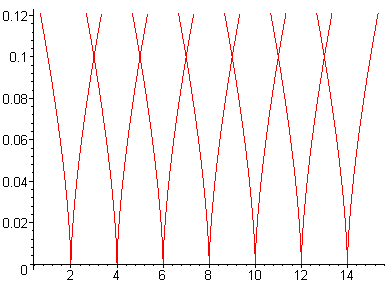ROV20_6.MWS

Chapter 20. Some Classes of Surfaces

> restart:

20.1 Algebraic Surfaces

Exercise .

1. Prove that the following surfaces are algebraic:

c) The swallow-tail, (fifth order)

> plot3d([u, -4*v^3-2*u*v, 3*v^4+u*v^2], u=-4..4,v=-1..1, axes=framed);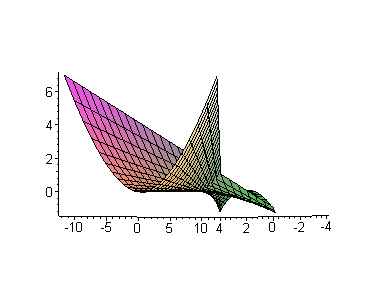d) The "wings"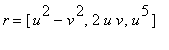, (tenth order).

Solution .

c) Using the resultant (the {resultant of two polynomials}) where x=u, we eliminate the variable v from the system of

equations -4v^3-2xv-y= 0, 3v^4+xv^2-z= 0 and obtain a polynomial in x,y,z of fifth order:

> F:=resultant(-4*v^3-2*x*v-y, 3*v^4+x*v^2-z, v);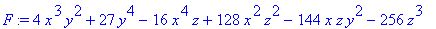d) Using twice the resultant we eliminate the variables u,v from the system of polynomial equations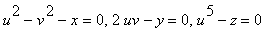, and obtain a polynomial in x,y,z of tenth order:

> res1:=resultant(u^2-v^2-x, 2*u*v-y, v);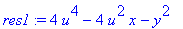> F:=resultant(res1, u^5-z, u);2.

a) The surface swallow-tail

> d:=discrim(t^4+x*t^2+y*t+z, t);> subs({y=-4*t^3-2*x*t,z=3*t^4+x*t^2}, d);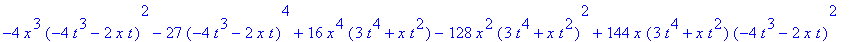> simplify(%); # checking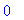c)

> F:=t^4+x*t^2+y*t+z: F1:=diff(F,t): F2:=diff(F1,t):

> sol:=solve({F,F1,F2}, {x,y,z});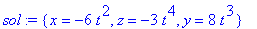> plots[spacecurve]([-6*t^2, 8*t^3, -3*t^4], t=-1..1, axes=boxed, thickness=2);>

20.2 Surfaces of Revolution

The surface of revolution of the curve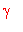(u):about the axis OZ is given in Figure together

with its parallels and meridians.

> plot3d([(4+sin(2*u))*cos(v), (4+sin(2*u))*sin(v), u], u=0..4*Pi, v=0..2*Pi, scaling=constrained, axes=framed);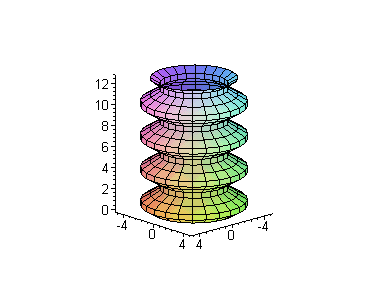The reader can use the following program to plot various surfaces of revolution and their profile curves in rotation.

> with(plots):

```Warning, existing definition for changecoords has been overwritten
```

> phi:=u -> cos(u): psi:=u->sin(u): n:=6: u0:=-Pi/2: u1:=Pi/2: # enter your data

> X:= phi(u)*cos(v): Y:= phi(u)*sin(v): Z:=psi(u):

> plot([phi(u), psi(u), u=u0..u1], scaling=constrained); # curve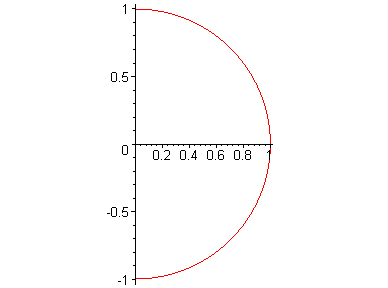> a:=k-> spacecurve([phi(u)*cos(k), phi(u)*sin(k),psi(u)], u=u0..u1, thickness=3, color=green): # profile curve

> b:=plot3d([X,Y,Z], u=u0..u1, v=-Pi..Pi, style=wireframe): # surface

> display([seq(display([a(k*2*Pi/n), b]), k=1..n)], insequence=true,scaling=constrained); # surface with rotating profile curve>

For example, in the case of revolution of the astroid (which is an algebraic curve of the sixth order), we eliminate

r from the system of equations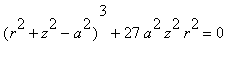,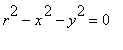and obtain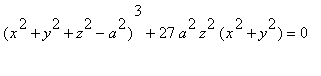, i.e., a surface of sixth order.

> restart: resultant(r^2-x^2-y^2, (r^2+z^2-a^2)^3+27*a^2*z^2*r^2, r);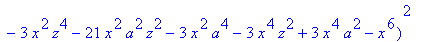For example, in the case of revolution of a circle, we eliminate r from the system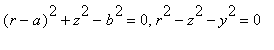(with parameters a>b>0) and obtain a polynomial that defines a torus of revolution:

> resultant((r-a)^2+z^2-b^2, r^2-x^2-y^2, r);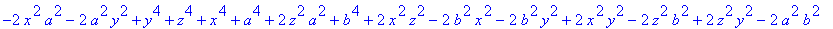which (check using Maple ) can be simplified to the short form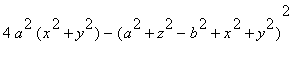.

5. Seven colors are sufficient to color a chart on a torus (see the problem of four colors [Gar 2]).

Plot it using animation as in the following program.

> with(plots): setoptions3d(style=patchnogrid, projection=0.5, orientation=[40,50], scaling=constrained):

```Warning, existing definition for changecoords has been overwritten
```

> r1:=3: r2:=1: G:=array(0..14):

> xk:=(r1+r2*sin(u))*cos(v+2*Pi*k/7): yk:=(r1+r2*sin(u))*sin(v+2*Pi*k/7): zk:=r2*cos(u):

> for i from 1 to 7 do G[i]:=k->plot3d([xk,yk,zk], u=0..2*Pi, v=(2*Pi*(2*i-2)+5*u)/14..(2*Pi*(2*i)+5*u)/14, color=(i-1)/7, grid=[30,10]): G[7+i]:=k->spacecurve([subs(v=(2*Pi*(2*i-2)+5*u)/14,xk), subs(v=(2*Pi*(2*i-2)+5*u)/14, yk), subs(v=(2*Pi*(2*i-2)+5*u)/14, zk), u=0..2*Pi], thickness=3, color=black, numpoints=30) od:

> G:=k->spacecurve([subs(u=2*Pi, xk), subs(u=2*Pi, yk), subs(u=2*Pi, zk)], thickness=3, v=0..2*Pi, color=black, numpoints=30):

> display([seq(display([seq(G[i](k), i=0..14)]), k=1..7)], insequence=true);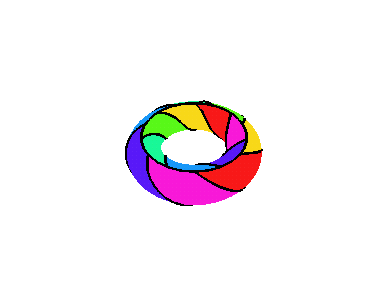The 2-dimensional chart (scheme) is the following:

> G2:=array(0..9): for i from 1 to 9 do G2[i]:=plot([u+2*i-6,u,u=0..5], view=[0..14,0..5]) od:

> G2:=polygonplot([[0,0], [14,0], [14,5], [0,5]]):

> plots[display]([seq(G2[i], i=0..9)], scaling=constrained);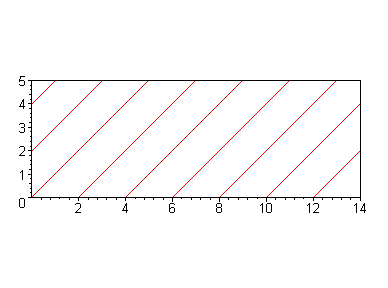6. Slowly changing the equations of a torus (using periodic flattening of the circle to a line segment) leads to

a {pinched torus}:

> plot3d([(1+0.2*sin(u))*cos(v), (1+0.2*sin(u))*sin(v),0.2*sin(v)*cos(u)], v=0..2*Pi, u=0..2*Pi,style=patch);9. The Mobius band

> plot3d([(5+sin(v/2)*u)*cos(v), (5+sin(v/2)*u)*sin(v),cos(v/2)*u], v=0..2*Pi, u=-1..1, grid=[60,10],scaling=CONSTRAINED, orientation=[-106,70],style=patch);>

The n-times turned (through 180^0) twisted strip

> n:=3: plot3d([(5+u*sin(n*v/2))*cos(v), (5+u*sin(n*v/2))*sin(v), u*cos(n*v/2)], v=0..2*Pi, u=-1..1, grid=[60,10], scaling=CONSTRAINED, orientation=[-106,70], style=patch);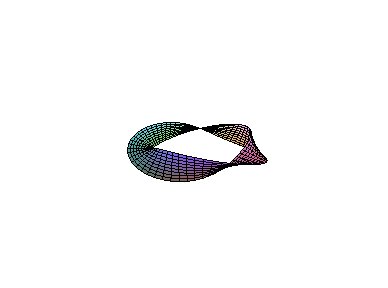>

20.3 Ruled Surfaces

20.3.1 Some Types of Ruled Surfaces

7. The program and plot the normal surface and the binormal surface r_1(u,v)=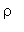(u)+v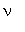(u), r_2(u,v)=(u)+v(u),

The intermediate surfaces for some values of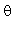:(u,v)=(u)+v cos()(u)+v sin()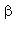(u).

> restart: with(plots): with(linalg):

```Warning, existing definition for changecoords has been overwritten
```

```Warning, protected name norm has been changed and unprotected
```

```Warning, protected name trace has been changed and unprotected
```

> r:=array([cos(u)^2, cos(u)*sin(u), sin(u)]);> spacecurve(r, u=-Pi..Pi, thickness=3); # Viviani, Fig. 20.28}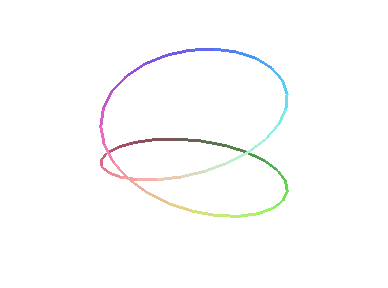> p1:=plot3d([cos(u)*cos(v), cos(u)*sin(v), sin(u)],u=-Pi/2..Pi/2, v=0..2*Pi, grid=[30,60], style=patch):

> p2:=plot3d([0.5+cos(u)/2, sin(u)/2,v],v=-1.2..1.2, u=0..2*Pi, grid=[20,45]):

> display3d({p1, p2},scaling=constrained); # Viviani as intersection> ru:=map(diff,r,u); ruu:=map(diff,ru,u);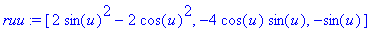> tt:=scalarmul(ru, 1/norm(ru,2)):

> b:=crossprod(ru, ruu): bb:=scalarmul(b, 1/norm(b,2)):

> nn:=crossprod(bb, tt): rn:=evalm(r+scalarmul(nn, v)):

> rt:=evalm(r+scalarmul(tt, v)): rb:=evalm(r+scalarmul(bb, v)):

> rtheta:=evalm(r+scalarmul(nn, v*cos(Pi/6))+scalarmul(bb, v*cos(Pi/6))):

> plot3d(rt, u=-Pi..Pi, v=-1.8..1.8, grid=[70,20],scaling=constrained);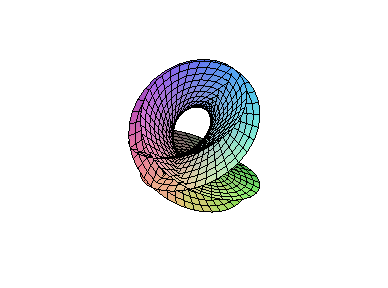> plot3d(rn, u=-Pi..Pi, v=-1..1, grid=[70,20], scaling=constrained);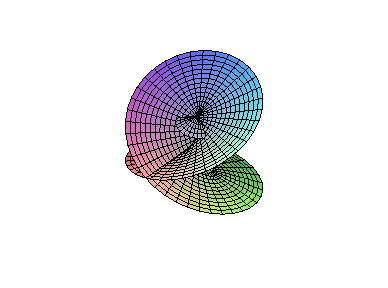> plot3d(rb, u=-Pi..Pi,v=-0.5..0.5, grid=[70,15], scaling=constrained);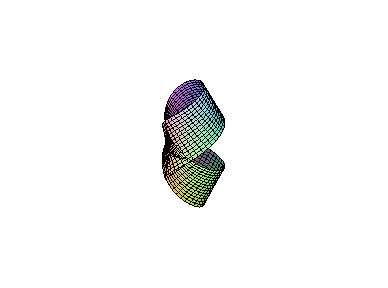> plot3d(rtheta, u=-Pi..Pi,v=-2..2, grid=[70,20], scaling=constrained);>

20.3.2 Striction Curve and Distribution Parameter

3. Calculate using Maple the striction curve and the distribution parameter of the hyperbolic paraboloid.

> restart: with(linalg): d:=evalm([0, 1, a*u]/sqrt(1+(a*u)^2)):

```Warning, protected name norm has been changed and unprotected
```

```Warning, protected name trace has been changed and unprotected
```

> r:=vector([u,0,0]): ru:=map(diff, r, u): du:=map(diff, d, u);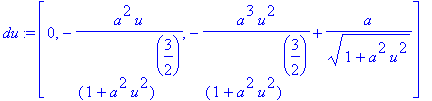> p(u):=simplify(det(matrix(3,3, [d,du,ru]))/dotprod(du, du, orthogonal));>

20.4 Envelope of a One-Parameter Family of Surfaces

20.4.1 Theorem on an Envelope of Surfaces

Example 4 .

> for t from 1 to 6 do p[t]:=plot3d([.8*t+cos(u)*cos(v), cos(u)*sin(v), sin(u)], u=-Pi/2..Pi/2, v=0..2*Pi) od:

> p:=plot3d([v, cos(u), sin(u)], u=-Pi-1..0, v=-0.5..6):

> plots[display]([seq(p[t], t=1..7)], scaling=constrained);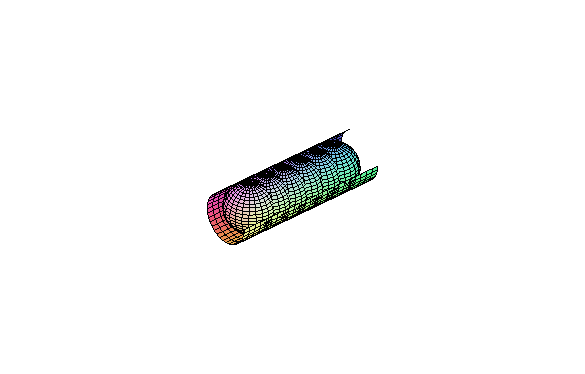The envelope from Example 20.4.2:

> for t from 1 to 12 do p[t]:=plot3d([3*cos(t*Pi/6)+cos(u)*cos(v), 3*sin(t*Pi/6)+cos(u)*sin(v), sin(u)], u=-Pi/2..Pi/2, v=0..2*Pi) od:

> p:=plot3d([(3+cos(u))*cos(v), (3+cos(u))*sin(v),sin(u)], u=-Pi-1.3..0.7, v=Pi/3..2*Pi):

> plots[display]([seq(p[t], t=1..13)], scaling=constrained);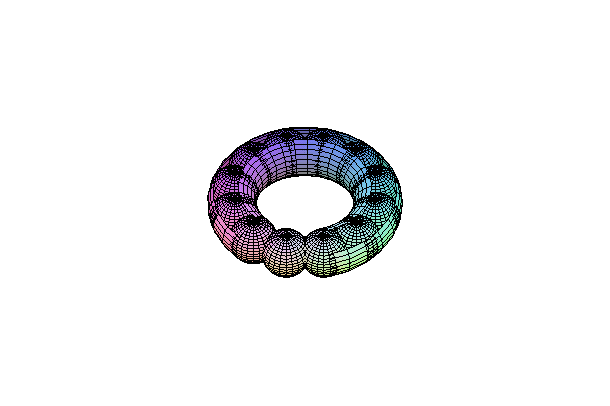>

The discriminant set of the familyof cylindrical surfaces with

the axis OZ coincides with a plane {y=0}.

> p:=t-> plot3d([u^3+2*t, u^2/10, v], u=-1.5..1.5, v=0..0.1, grid=[60,10]): plots[display]([seq(p(t), t=1..7)], scaling=unconstrained);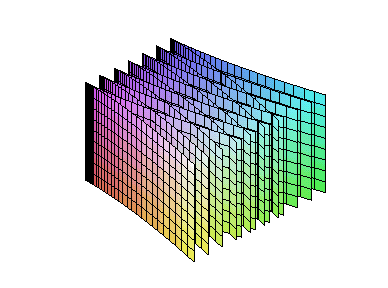> p:=t->plot([u^3+2*t, u^2/10, u=-1.1..1.1]): plots[display]([seq(p(t), t=1..7)]);